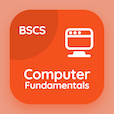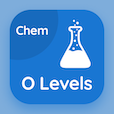Computer Science Online Courses

Computer Basics Quizzes

Computer Basics Quiz PDF - Complete

# Binary Coded Decimal Quiz MCQ Online p. 63

Practice Binary Coded Decimal quiz questions and answers PDF, binary coded decimal trivia questions 63 to learn online Computer Basics course for online classes. Processing Data MCQ questions, Binary Coded Decimal Multiple Choice Questions (MCQ) for online college degrees. "Binary Coded Decimal Quiz" PDF eBook: iso and osi models, backup utilities, computer printers, output devices, binary coded decimal test prep for online computer science schools.

"Resultant binary of the decimal problem 49 + 1 is" MCQ PDF: 110011, 110010, 1010101, and 111100 for online software development courses. Solve processing data questions and answers to improve problem solving skills for computer software engineer.

## Trivia Quiz on Binary Coded Decimal MCQs

MCQ: Resultant binary of the decimal problem 49 + 1 is

110010
110011
1010101
111100

MCQ: Computer device which is used to displays output on a screen is

monitor
LCD
LED
projector

MCQ: Types of computer impact printer are

dot matrix printers
daisy wheel printers
line printers
all of these

MCQ: A deadlock exists in a system if and only if the wait for graph

has a cycle in it
has a path from first node to last node
is a tree
has a layout in it

MCQ: Layer of computer OSI model which performs data compression is

network
presentation
physical

### More Quizzes from Computer Basics Course